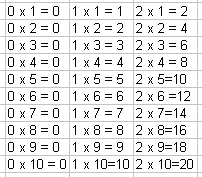Name: ___________________Date:___________________

 Email us to get an instant 20% discount on highly effective K-12 Math & English kwizNET Programs!

### Grade 4 - Mathematics3.2 Multiplication Facts 0-5Directions: Review the time table and answer the following questions. Also write ten examples of your own and multiplication facts for 0,1,2,3,4,5 on a sheet of paper.
 Q 1: 3 x 3 =690 Q 2: 11 x 8 =882011 Q 3: 4 x 8=323624 Q 4: 12 x 2112624 Q 5: 3 x 9 =282627 Q 6: 5 x 4=91120 Q 7: 4 x 9=362813 Q 8: 2 x 8 =41610 Question 9: This question is available to subscribers only! Question 10: This question is available to subscribers only!